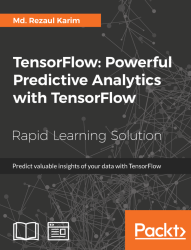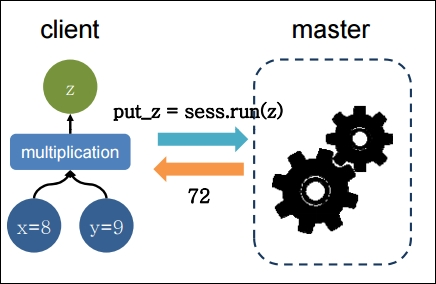•#### TensorFlow: Powerful Predictive Analytics with TensorFlow#### Overview of this book

Predictive analytics discovers hidden patterns from structured and unstructured data for automated decision making in business intelligence. Predictive decisions are becoming a huge trend worldwide, catering to wide industry sectors by predicting which decisions are more likely to give maximum results. TensorFlow, Google’s brainchild, is immensely popular and extensively used for predictive analysis. This book is a quick learning guide on all the three types of machine learning, that is, supervised, unsupervised, and reinforcement learning with TensorFlow. This book will teach you predictive analytics for high-dimensional and sequence data. In particular, you will learn the linear regression model for regression analysis. You will also learn how to use regression for predicting continuous values. You will learn supervised learning algorithms for predictive analytics. You will explore unsupervised learning and clustering using K-meansYou will then learn how to predict neighborhoods using K-means, and then, see another example of clustering audio clips based on their audio features. This book is ideal for developers, data analysts, machine learning practitioners, and deep learning enthusiasts who want to build powerful, robust, and accurate predictive models with the power of TensorFlow. This book is embedded with useful assessments that will help you revise the concepts you have learned in this book. This book is repurposed for this specific learning experience from material from Packt's Predictive Analytics with TensorFlow by Md. Rezaul Karim.
TensorFlow: Powerful Predictive Analytics with TensorFlowCreditsPrefaceFree Chapter
From Data to Decisions – Getting Started with TensorFlowPutting Data in Place – Supervised Learning for Predictive AnalyticsClustering Your Data – Unsupervised Learning for Predictive AnalyticsUsing Reinforcement Learning for Predictive Analytics## TensorFlow Programming Model

The TensorFlow programming model signifies how to structure your predictive models. A TensorFlow program is generally divided into four phases once you have imported TensorFlow library for associated resources:

• Construction of the computational graph that involves some operations on tensors (we will see what is a tensor soon)

• Create a session

• Running a session, that is performed for the operations defined in the graph

• Computation for data collection and analysis

These main steps define the programming model in TensorFlow. Consider the following example, in which we want to multiply two numbers:

```import tensorflow as tf
x = tf.constant(8)
y = tf.constant(9)
z = tf.multiply(x, y)
sess = tf.Session()
out_z = sess.run(z)
Finally, close the TensorFlow session when you're done:
sess.close()print('The multiplicaiton of x and y: %d' % out_z)```

The preceding code segment can be represented by the following figure:Figure 8: A simple multiplication executed and returned the product on client-master architecture

To make the preceding program more efficient, TensorFlow also allows you to exchange data in your graph variables through placeholders (to be discussed later). Now imagine the following code segment that does the same but in a more efficient way:

```# Import tensorflow
import tensorflow as tf
# Build a graph and create session passing the graph:
with tf.Session() as sess:
x = tf.placeholder(tf.float32, name="x")
y = tf.placeholder(tf.float32, name="y")
z = tf.multiply(x,y)
# Put the values 8,9 on the placeholders x,y and execute the graph
z_output = sess.run(z,feed_dict={x: 8, y:9})
# Finally, close the TensorFlow session when you're done:
sess.close()
print(z_output)```

TensorFlow is not necessary to multiply two numbers; also the number of lines of the code for this simple operation is so many. However, the example wants to clarify how to structure any code, from the simplest as in this instance, to the most complex. Furthermore, the example also contains some basic instructions that we will find in all the other examples given in the course of this book.

### Note

We will demonstrate most of the examples in this book with Python 3.x compatible. However, a few examples will be given using Python 2.7.x too.

This single import in the first line helps to import the TensorFlow for your command that can be instantiated with `tf as stated earlier. Then the `TensorFlow operator will then be expressed by `tf` and the dot '`.`' and by the name of the operator to use. In the next line, we construct the object `session`, by means of the instruction `tf.Session()`:

`with tf.Session() as sess:`

### Note

The session object (that is, sess) encapsulates the environment for the TensorFlow so that all the operation objects are executed, and Tensor objects are evaluated. We will see them in upcoming sections.

This object contains the computation graph, which as we said earlier, are the calculations to be carried out.

The following two lines define variables x and y, using the notion of placeholder. Through a placeholder you may define both an input (such as the variable x of our example) and an output variable (such as the variable y):

```x = tf.placeholder(tf.float32, name='x')
y = tf.placeholder(tf.float32, name='y')```

Placeholder provides an interface between the elements of the graph and the computational data of the problem, it allows us to create our operations and build our computation graph, without needing the data, but only a reference to it.

To define a data or tensor (soon I will introduce you to the concept of tensor) via the placeholder function, three arguments are required:

• Data type: Is the type of element in the tensor to be fed.

• Shape: Of the placeholder–that is, shape of the tensor to be fed (optional). If the shape is not specified, you can feed a tensor of any shape.

• Name: Very useful for debugging and code analysis purposes, but it is optional.

### Note

For more, refer to https://www.tensorflow.org/api_docs/python/tf/Tensor.

So, we may introduce the model that we want to compute with two arguments, the placeholder and the constant that are previously defined. Next, we define the computational model.

The following statement, inside the session, builds the data structures of the `x` product with `y`, and the subsequent assignment of the result of the operation to the placeholder `z`. Then it goes as follows:

`  z = tf.multiply(x, y)`

Now since the result is already held by the placeholder `z`, we execute the graph, through the `sess.run`statement. Here we feed two values to patch a tensor into a graph node. It temporarily replaces the output of an operation with a tensor value (more in upcoming sections):

`z_output = sess.run(z,feed_dict={x: 8, y:9})`

Then we close the TensorFlow session when we're done:

`sess.close()`

In the final instruction, we print out the result:

`     print(z_output)`

This essentially prints output 72.0.